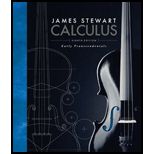# As in Exercise 9, consider a spring with mass m, spring constant k , and damping constant c = 0, and let ω = k / m . If an external force F ( t ) = F 0 cos ωt is applied (the applied frequency equals the natural frequency), use the method of undetermined coefficients to show that the motion of the mass is given by x ( t ) = c 1 cos ω t + c 2 sin ω t + F 0 2 m ω t sin ω t### Calculus: Early Transcendentals

8th Edition
James Stewart
Publisher: Cengage Learning
ISBN: 9781285741550### Calculus: Early Transcendentals

8th Edition
James Stewart
Publisher: Cengage Learning
ISBN: 9781285741550

#### Solutions

Chapter
Section
Chapter 17.3, Problem 10E
Textbook Problem

## Expert Solution

### Want to see the full answer?

Check out a sample textbook solution.See solution

### Want to see this answer and more?

Experts are waiting 24/7 to provide step-by-step solutions in as fast as 30 minutes!*

See Solution

*Response times vary by subject and question complexity. Median response time is 34 minutes and may be longer for new subjects.